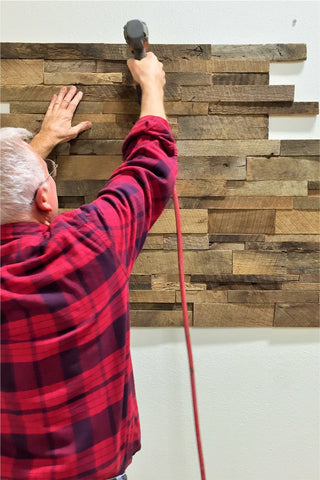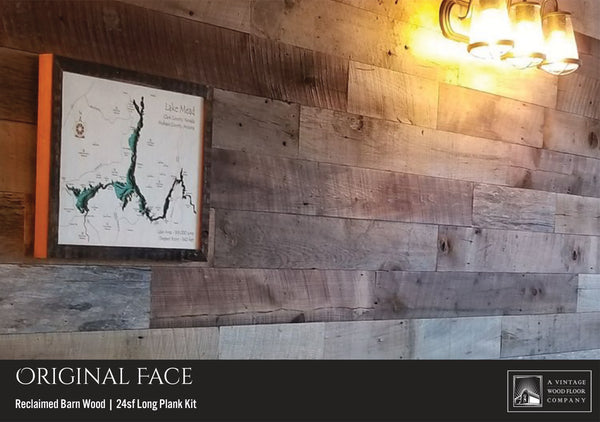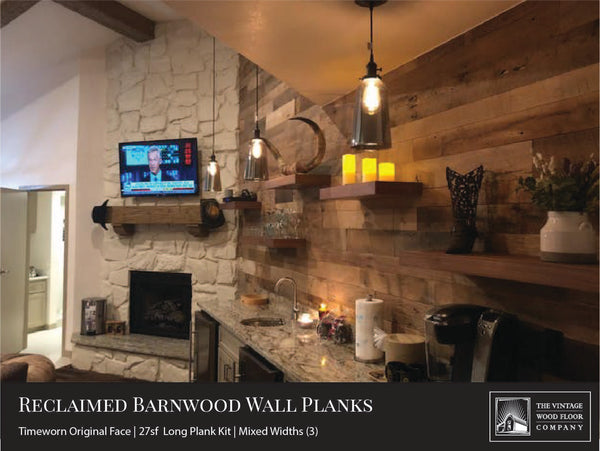# How Many Wood Wall Panels Do I Need?Note: Each of our reclaimed wood wall panels covers 3 square feet
1. Measure the wall or area you want to cover (length X height) in feet
2. Divide this value by 3 = the number of panels you need

EXAMPLE: 3ft x 5ft wall/area needing coverage

• My area measures 3ft x 5ft = 15sf
• Coverage I need is 15sf
• The Math: 15sf (coverage needed) / 3sf (this is the panel coverage) = 5 panels needed (one 5-panel box)

NOTE: We suggest ordering 10%-15% extra to ensure you allow for waste and errors when installing. Begin by order as many boxes you need (5-panel box) then add single panels to your order for incremental coverage (e.g. if you need 11 panels, you should order 2 5-panel boxes and 1 single panel.

Do not order only single panels, as they cost more than the 5-panel box).## How Many 24sf Reclaimed Barn Wood Wall Plank Kits Do I Need?Calculate In Inches: Each kit covers 24 square feet (up to 8 foot lengths)
1. Measure the wall or area you want to cover (length X height) in inches
2. Divide this value by 12 to get the square feet
3. Divide this value by 24 = the number of kits you need

EXAMPLE: 36" x 96" wall/area needing coverage

• My area measures 36" x 96" = 3,456 square inches
• Divide 3,456 by 12 to get square feet = 288 square feet
• Divide 288 by 24 to get the number of kits needed = 12
• I need 12 kits
• The Math: 288sf (coverage needed) / 24sf (this is the kit's coverage) = 12 kits
Calculate In Feet: Each kit covers 24 square feet
1. Measure the wall or area you want to cover (length X height) in feet
2. Divide this value by 12 to get the square feet
3. Divide this value by 24 = the number of kits you need

EXAMPLE: 3ft x 8ft wall/area needing coverage

• My area measures 3ft x 8ft = 24 square feet
• Divide 24 by 24 to get the number of kits needed = 1
• I need 1 kit
• The Math: 24sf (coverage needed) / 24sf (this is the kit's coverage) = 1 kit

NOTE: We suggest ordering 10%-15% extra to ensure you allow for waste and errors when installing. Begin by order as many kit boxes you need

## How Many 27sf Reclaimed Barn Wood Wall Plank Kits Do I Need?This formula calculates 24sf to include 3sf extra for cuts and waste.
Calculate In Inches: Each kit covers 27 square feet (up to 6 foot lengths)
1. Measure the wall or area you want to cover (length X height) in inches
2. Divide this value by 12 to get the square feet
3. Divide this value by 24 = the number of kits you need which will leave some extra for cuts and waste

EXAMPLE: 36" x 96" wall/area needing coverage

• My area measures 36" x 96" = 3,456 square inches
• Divide 3,456 by 12 to get square feet = 288 square feet
• Divide 288 by 24 to get the number of kits needed = 12
• I need 12 kits
• The Math: 288sf (coverage needed) / 24sf (this is the kit's minimum coverage) = 12 kits
Calculate In Feet: Each kit covers 27 square feet but this calculation is to include extra for cuts and waste.
1. Measure the wall or area you want to cover (length X height) in feet
2. Divide this value by 12 to get the square feet
3. Divide this value by 24 = the number of kits you need

EXAMPLE: 3ft x 8ft wall/area needing coverage

• My area measures 3ft x 8ft = 24 square feet
• Divide 24 by 24 to get the number of kits needed = 1
• I need 1 kit
• The Math: 24sf (coverage needed) / 24sf (this is the kit's minimum coverage) = 1 kit

NOTE: We suggest ordering 10%-15% extra to ensure you allow for waste and errors when installing. This calculation includes this cuts and waste factor.